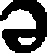# empirical formula

(redirected from empirical formulae)
Also found in: Dictionary, Thesaurus, Encyclopedia.

## formula

[for´mu-lah] (pl. formulas, for´mulae) (L.)
1. an expression, using numbers or symbols, giving the directions for preparing a compound (such as a medicine) or giving a procedure to follow to obtain a desired result.
2. a mixture for feeding an infant, usually with cow's milk as a base, supplemented with vitamins and minerals. Various formulas are available, differing in protein, fat, and carbohydrate content in order to meet the nutritional requirements or restrictions of individual infants.
chemical formula a combination of symbols used to express the chemical components of a substance.
empirical formula a chemical formula that expresses the proportions of the elements present in a substance.
molecular formula a chemical formula expressing the number of each element present in a substance, without indicating how they are linked.
spatial formula (stereochemical formula) a chemical formula giving the numbers of atoms of each element present in a molecule of a substance, which atom is linked to which, the types of linkages involved, and the relative positions of the atoms in space.
structural formula a chemical formula showing the spatial arrangement of the atoms and the linkage of every atom.

## empirical formula

a chemical formula that shows the smallest whole number ratio of atoms of different elements in a molecule. It does not indicate structural linkage. An example is CH2O, a carbohydrate.

## empirical formula (em·piˑ·r·kl fōrˑ·myōō·l),

n the simplest whole-number formula used to express the composition of a chemical compound.

## formula

pl. formulae, formulas [L.] an expression, using numbers or symbols, of the composition of, or of directions for preparing, a compound, such as a medicine, or of a procedure to follow to obtain a desired result, or of a single concept.

chemical formula
a combination of symbols used to express the chemical components of a substance.
dental formula
see dental formula.
empirical formula
a chemical formula that expresses the proportions of the elements present in a substance.
gait formula
sets out the times that the feet are individually in contact with the ground while the animal is moving.
molecular formula
a chemical formula expressing the number of atoms of each element present in a substance, without indicating how they are linked.
spatial formula, stereochemical formula
a chemical formula giving the numbers of atoms of each element present in a molecule of a substance, which atom is linked to which, the types of linkages involved, and the relative positions of the atoms in space.
structural formula
a chemical formula showing the spatial arrangement of the atoms and the linkage of every atom.
vertebral formula
sets out the number of vertebrae in each of the sections of the spinal column.
References in periodicals archive ?
Envelope curves for maximum floods of the Indus and Jhelum river basins can be used for preliminary flood estimation studies in these river basins because it seems to provide more realistic discharge estimation as compare to empirical formulae which are applicable only for small catchments.
We also indicate that if the substrate is changed, the above empirical formulae should be renewed just according to the presented procedures.
The empirical formulae of creep compliance can be obtained by applying the modeling approaches described earlier to the test data obtained.

Site: Follow: Share:
Open / Close